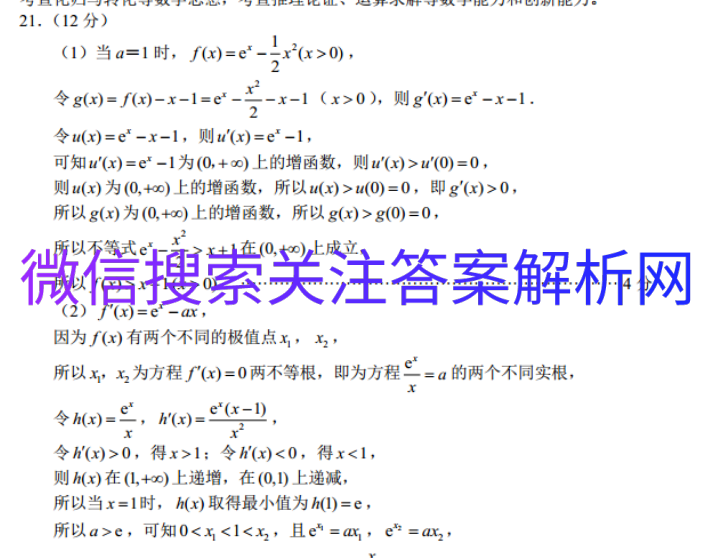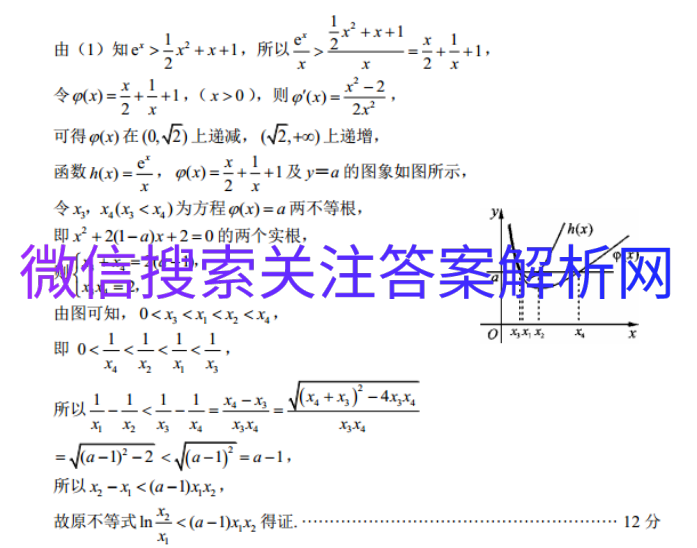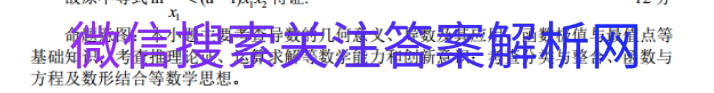# 全国100所名校最新高考模拟示范卷理科诶

2021-04-03 12:4821.(12分)(1)当a=1时,f(x)=e2-x2(x>0),令g(x)=f(x)-x-1=c2~x-1(x>0),则g'(x)=e-x-1令u(x)=e-x-1,则u(x)=e-1,可知u'(x)=e2-1为(0,+∞)上的增函数,则u(x)>a(O)=0,则a(x)为(0,+∞)上的增函数,所以u(x)>(0)=0,即g(x)>0,所以g(x)为(0,+∞)上的增函数,所以g(x)>g(O)=0x2所以不等式e->x+1在(0,+∞)上成立所以f(x)>x+1(x>0)(2)f(x)因为f(x)有两个不同的极值点x,x2所以xx2为方程f(x)=0两不等根,即为方程一=a的两个不同实根,令h(e,(x)e(x-,令h(x)>0,得x>1:令h(x)<0,得x<1则h(x)在(1,+∞)上递增,在(O,1)上递减所以当x=1时,M(x)取得最小值为h()=e,所以a>e,可知0 x2+x+1,所、x2+x+1令叫(x)=+-+1,(x>0),则(x)可得(x)在(√上递减,(2,+x)上递增,函数M(x)=,()=+1+1及y=a的图象如图所示,令xy,x(x3